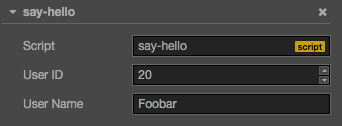# 使用 cc.Class 声明类型

`cc.Class` 是一个很常用的 API，用于声明 Cocos Creator 中的类，为了方便区分，我们把使用 cc.Class 声明的类叫做 CCClass

## 定义 CCClass

``````var Sprite = cc.Class({
name: "sprite"
});
``````

## 实例化

`Sprite` 变量保存的是一个 JavaScript 构造函数，可以直接 new 出一个对象：

``````var obj = new Sprite();
``````

## 判断类型

``````cc.log(obj instanceof Sprite);       // true
``````

## 构造函数

``````var Sprite = cc.Class({
ctor: function () {
cc.log(this instanceof Sprite);    // true
}
});
``````

## 实例方法

``````var Sprite = cc.Class({
// 声明一个名叫 "print" 的实例方法
print: function () { }
});
``````

## 继承

``````// 父类
var Shape = cc.Class();

// 子类
var Rect = cc.Class({
extends: Shape
});
``````

### 父构造函数

``````var Shape = cc.Class({
ctor: function () {
cc.log("Shape");    // 实例化时，父构造函数会自动调用，
}
});

var Rect = cc.Class({
extends: Shape
});

var Square = cc.Class({
extends: Rect,
ctor: function () {
cc.log("Square");   // 再调用子构造函数
}
});

var square = new Square();
``````

## 声明属性

``````cc.Class({
extends: cc.Component,
properties: {
userID: 20,
}
});
``````### 简单声明

• 当声明的属性为基本 JavaScript 类型时，可以直接赋予默认值：

``````  properties: {
height: 20,       // number
type: "actor",    // string
target: null,     // object
}
``````
• 当声明的属性具备类型时（如：`cc.Node``cc.Vec2` 等），可以在声明处填写他们的构造函数来完成声明，如：

``````  properties: {
target: cc.Node,
pos: cc.Vec2,
}
``````
• 当声明属性的类型继承自 `cc.ValueType` 时（如：`cc.Vec2``cc.Color``cc.Rect`），除了上面的构造函数，还可以直接使用实例作为默认值：

``````  properties: {
pos: new cc.Vec2(10, 20),
color: new cc.Color(255, 255, 255, 128),
}
``````
• 当声明属性是一个数组时，可以在声明处填写他们的类型或构造函数来完成声明，如：

``````  properties: {
any: [],      // 不定义具体类型的数组
bools: [cc.Boolean],
strings: [cc.String],
floats: [cc.Float],
ints: [cc.Integer],

values: [cc.Vec2],
nodes: [cc.Node],
frames: [cc.SpriteFrame],
}
``````

### 完整声明

``````properties: {
score: {
default: 0,
displayName: "Score (player)",
tooltip: "The score of player",
}
}
``````

• default: 设置属性的默认值，这个默认值仅在组件第一次添加到节点上时才会用到
• type: 限定属性的数据类型，详见 CCClass 进阶参考：type 参数
• visible: 设为 false 则不在 属性检查器 面板中显示该属性
• serializable: 设为 false 则不序列化（保存）该属性
• displayName: 在 属性检查器 面板中显示成指定名字
• tooltip: 在 属性检查器 面板中添加属性的 Tooltip

#### 数组声明

``````properties: {
names: {
default: [],
type: [cc.String]   // 用 type 指定数组的每个元素都是字符串类型
},

enemies: {
default: [],
type: [cc.Node]     // type 同样写成数组，提高代码可读性
},
}
``````

### get/set 声明

``````properties: {
width: {
get: function () {
return this._width;
},
set: function (value) {
this._width = value;
}
}
}
``````